# Step-by-step Guide for Implementation of Hierarchical Clustering in R

## Recent Posts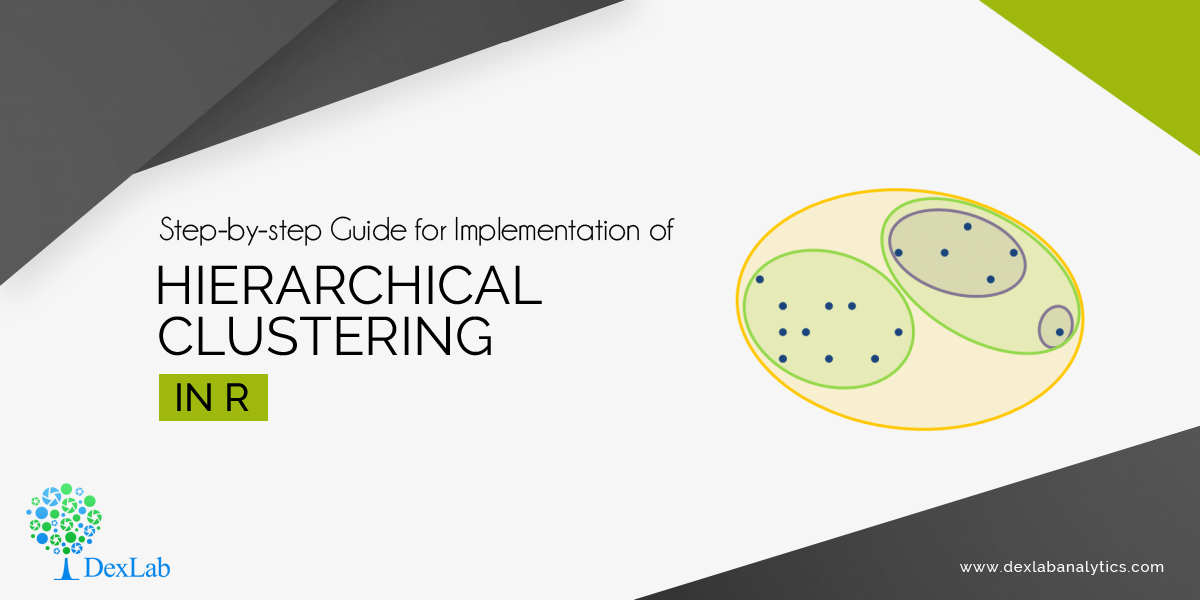Hierarchical clustering is a method of clustering that is used for classifying groups in a dataset. It doesn’t require prior specification of the number of clusters that needs to be generated. This cluster analysis method involves a set of algorithms that build dendograms, which are tree-like structures used to demonstrate the arrangement of clusters created by hierarchical clustering.

It is important to find the optimal number of clusters for representing the data. If the number of clusters chosen is too large or too small, then the precision in partitioning the data into clusters is low.

#### NbClust

The R package NbClust has been developed to help with this. It offers good clustering schemes to the user and provides 30 indices for determining the number of clusters.

Through NbClust, any combination of validation indices and clustering methods can be requested in a single function call. This enables the user to simultaneously evaluate several clustering schemes while varying the number of clusters.

One such index used for getting optimum number of clusters is Hubert Index.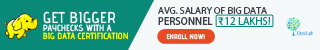#### Performing Hierarchical Clustering in R

In this blog, we shall be performing hierarchical clustering using the dataset for milk. The flexclust package is used to extract this dataset.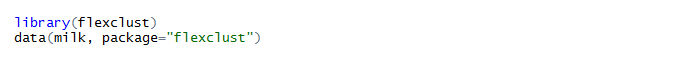The milk dataset contains observations and parameters as shown below: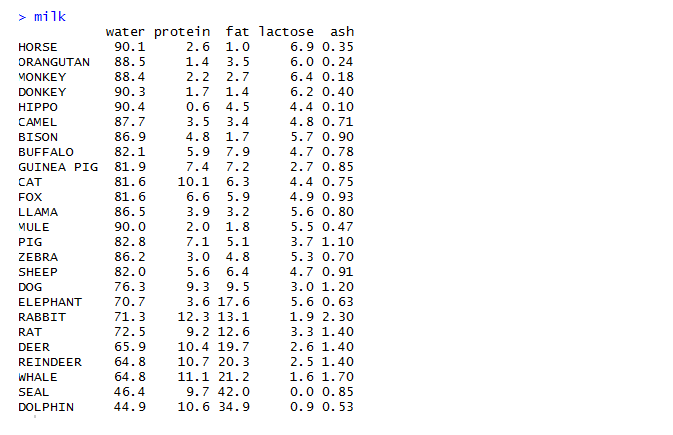As seen in the dataset, milk obtained from various animal sources and their respective proportions of water, protein, fat, lactose and ash have been mentioned.

For making calculations easier, we scale down original values into a standard normalized form. For that, we use processes like centering and scaling. The variable may be scaled in the following ways:

Subtract mean from each value (centering) and then divide it by standard deviation or divide it by its mean deviation about mean (scaling)

Divide each value in the variable by maximum value of the variable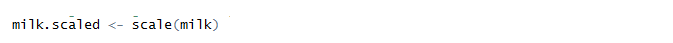After scaling the variables we get the following matrix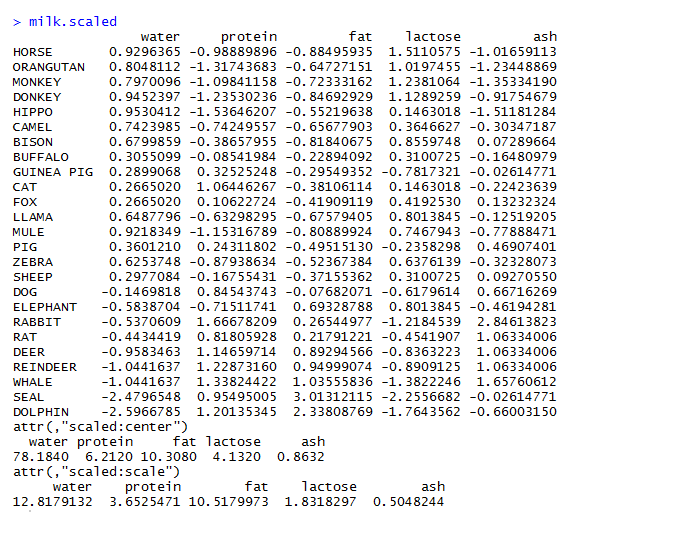The next step is to calculate the Euclidean distance between different data points and store the result in a variable.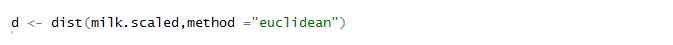Hierarchical average linkage method is used for performing clustering of different animal sources. The formula used for that is shown below.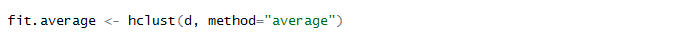We obtain 25 clusters from the dataset.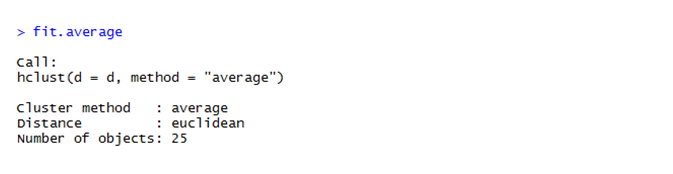To draw the dendogram we use the plot command and we obtain the figure given below.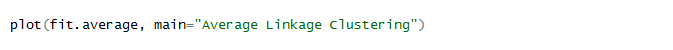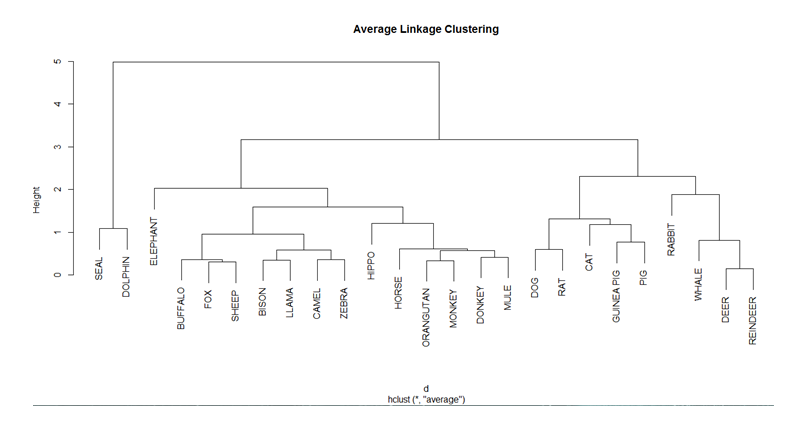The Nbclust library is used to get the optimum number of clusters for partitioning the data. The maximum and minimum number of clusters that is needed is stored in a variable. The nbClust method finds out the optimum number of clusters according to different clustering indices and finally the Hubert Index decides the optimum value of the number of clusters.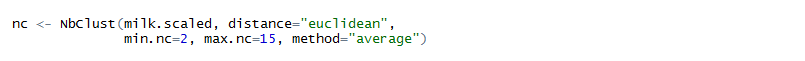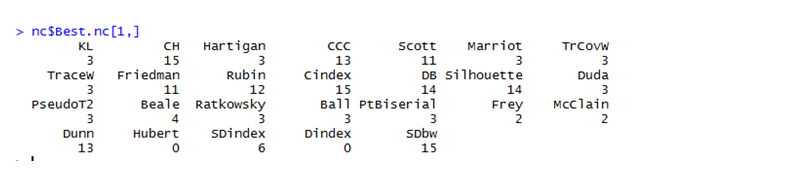The optimum cluster value is 3, as can be seen in the figure below.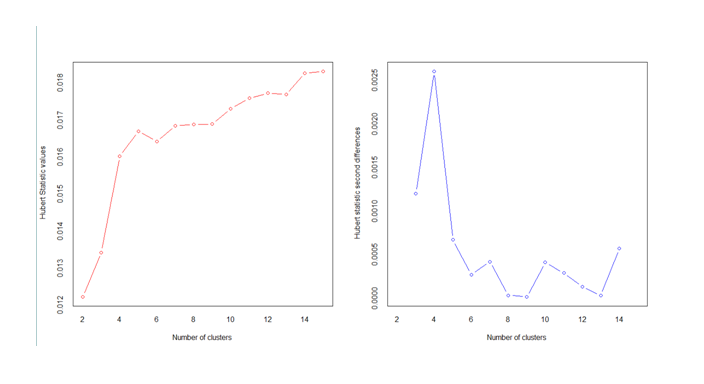Values corresponding to knee jerk visuals in the graph give the number of clusters needed.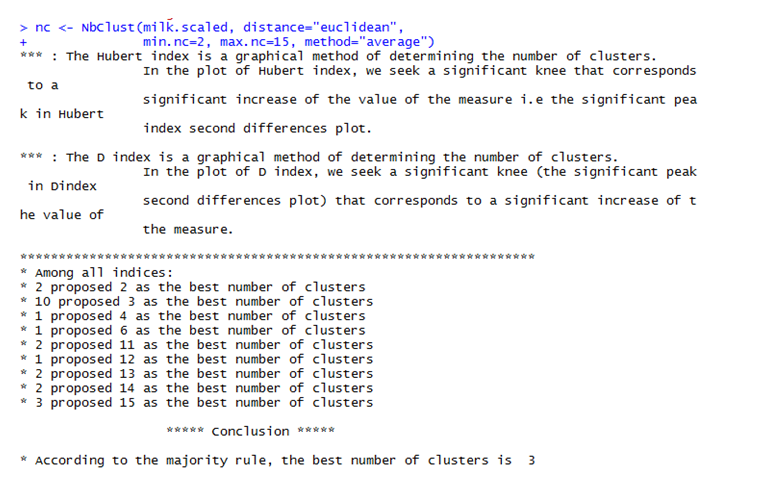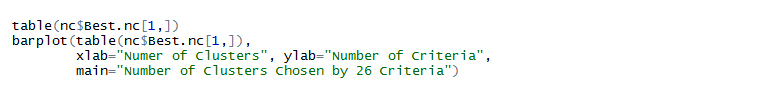The graph shows that the maximum votes from various clustering indices went to cluster 3. Hence, the data is partitioned into 3 clusters.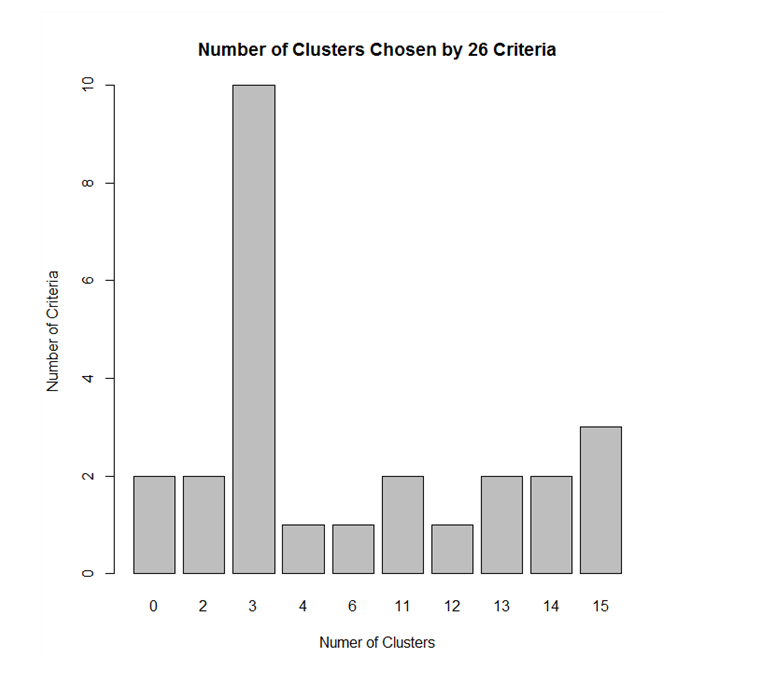The graph is partitioned into 3 clusters as shown by the red lines.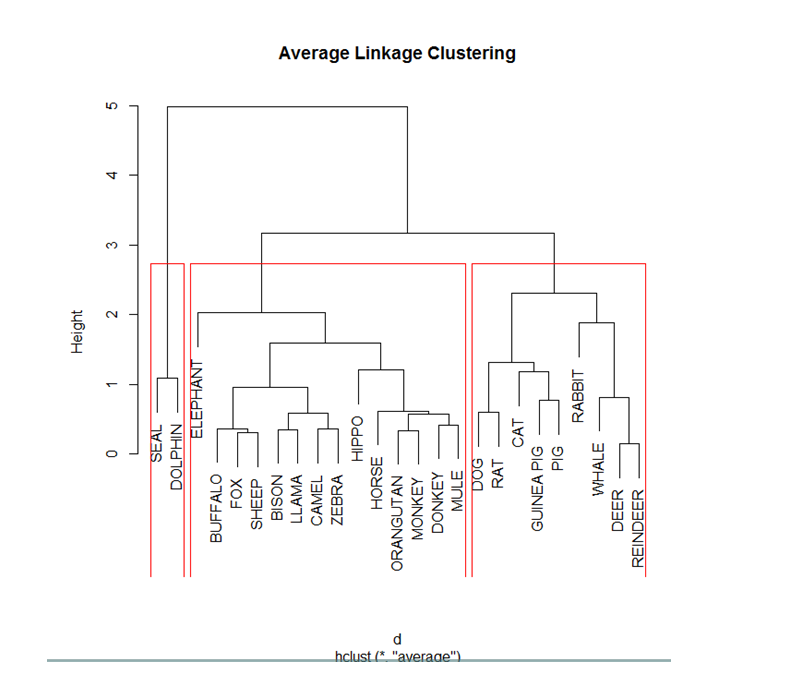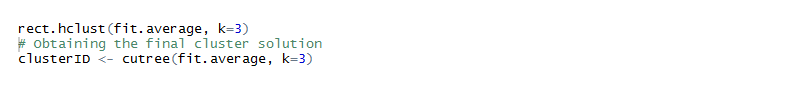Now, the points are portioned into 3 clusters as opposed to the 25 clusters we got initially.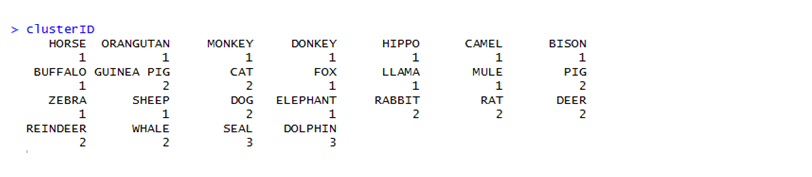Next, the clusters are assigned to the observations.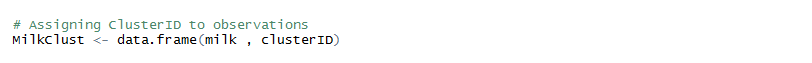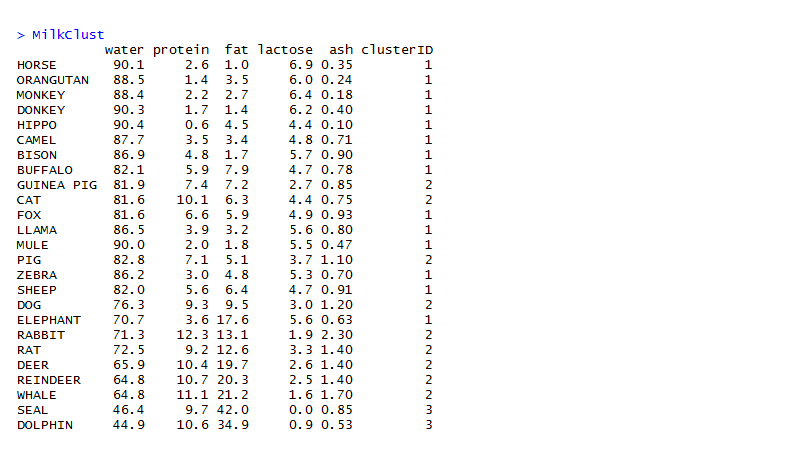The clusters are assigned different colors for ease of visualization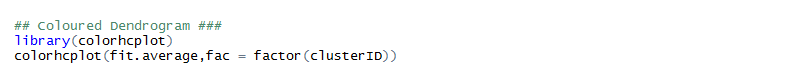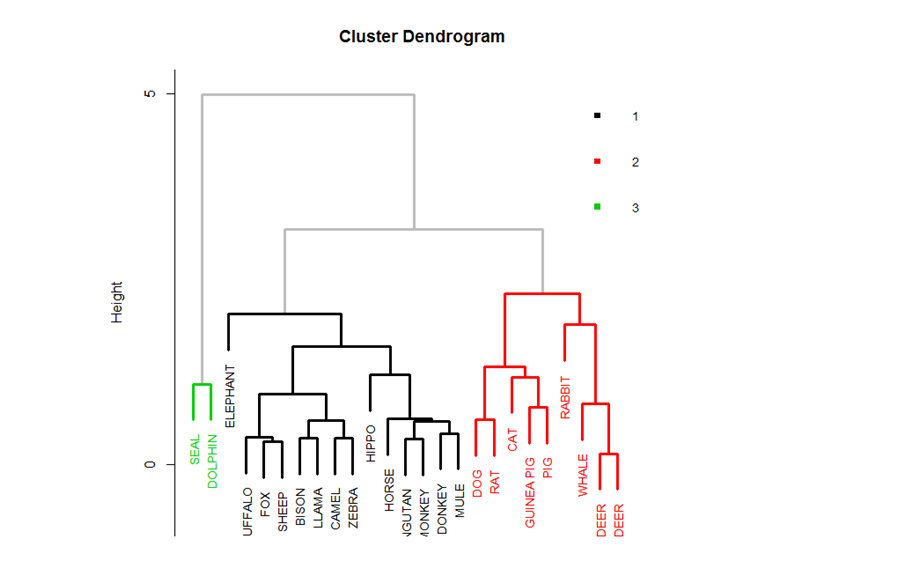That brings us to a close on the topic of Hierarchical clustering. In the upcoming blogs, we shall be discussing K-Means clustering. So, follow DexLab Analytics – a leading institute providing big data Hadoop training in Gurgaon. Enroll for their big data Hadoop courses and avail flat 10% discount. To more about this #SummerSpecial offer, visit our website.

#### Interested in a career in Data Analyst?### Call us to know more### Gurgaon+91 931 572 5902